# Test: Probability- 4

## 15 Questions MCQ Test Quantitative Aptitude for GMAT | Test: Probability- 4

Description
Attempt Test: Probability- 4 | 15 questions in 30 minutes | Mock test for GMAT preparation | Free important questions MCQ to study Quantitative Aptitude for GMAT for GMAT Exam | Download free PDF with solutions
QUESTION: 1

### A small, experimental plane has t hree engines, one of which is redundant. That is, as long as two of the engines are working, the plane will stay in the air. Over the course of a typical flight, there is a 1/3 chance that engine one will fail. There is a 75% probability that engine two will work. The third engine works only half the time. What is the probability that the plane will crash in any given flight?

Solution:

There are four scenarios in which the plane will crash. Determine the probability of each of these scenarios individually:
CASE ONE: Engine 1 fails, Engine 2 fails, Engine 3 works =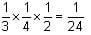CASE TWO: Engine 1 fails, Engine 2 works, Engine 3 fails =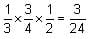CASE THREE: Engine 1 works, Engine 2 fails, Engine 3 fails =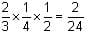CASE FOUR: Engine 1 fails, Engine 2 fails, Engine 3 fails =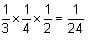To determine the probability that any one of these scenarios will occur, sum the four probabilities: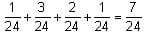QUESTION: 2

### Ms. Barton has four children. You are told correctly that she has at least two girls but you are not told which two of her four children are those girls. What is the probability that she also has two boys? (Assume that the probability of having a boy is the same as the probability of having a girl.)

Solution:

Since each of the 4 children can be either a boy or a girl, there are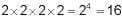possible ways that the children might be born, as listed below: BBBB (all boys) BBBG, BBGB, BGBB, GBBB, (3 boys, 1 girl) BBGG, BGGB, BGBG, GGBB, GBBG, GBGB (2 boys, 2 girls) GGGB, GGBG, GBGG, BGGG (3 girls, 1 boy) GGGG (all girls) Since we are told that there are at least 2 girls, we can eliminate 5 possibilities--the one possibility in which all of the children are boys (the first row) and the four possibilities in which only one of the children is a girl (the second row).
That leaves 11 possibilities (the third, fourth, and fifth row) of which only 6 are comprised of two boys and two girls (the third row). Thus, the probability that Ms. Barton also has 2 boys is 6/11.

QUESTION: 3

### Laura has a deck of standard playing cards with 13 of the 52 cards designated as a "heart." If Laura shuffles the deck thoroughly and then deals 10 cards off the top of the deck, what is the probability that the 10th card dealt is a heart?

Solution:

Although this may be counter-intuitive at first, the probability that any card in the deck will be a heart before any cards are seen is 13/52 or 1/4.
One way to understand this is to solve the problem analytically for any card by building a probability "tree" and summing the probability of all of its "branches."
For example, let's find the probability that the 2nd card dealt from the deck is a heart. There are two mutually exclusive ways this can happen: (1) both the first and second cards are hearts or (2) only the second card is a heart.
CASE 1: Using the multiplication rule, the probability that the first card is a heart AND the second card is a heart is equal to the probability of picking a heart on the first card (or 13/52, which is the number of hearts in a full deck divided by the number of cards) times the probability of picking a heart on the second card (or 12/51, which is the number of hearts remaining in the deck divided by the number of cards remaining in the deck).
13/52 x 12/51 = 12/204
CASE 2: Similarly, the probability that the first card is a non-heart AND the second card is a heart is equal to the probability that the first card is NOT a heart (or 39/52) times the probability of subsequently picking a heart on the 2nd card (or 13/51). 39/52 x 13/51 = 39/204
Since these two cases are mutually exclusive, we can add them together to get the total probability of getting a heart as the second card: 12/204 + 39/204 = 51/204 = 1/4.
We can do a similar analysis for any card in the deck, and, although the probability tree gets more complicated as the card number gets higher, the total probability that the n th card dealt will be a heart will always end up simplifying to 1/4.

QUESTION: 4

A license plate in the country Kerrania consists of four digits followed by two letters. The letters A, B, and C are used only by government vehicles while the letters D through Z are used by non-government vehicles. Kerrania's intelligence agency has recently captured a message from the country Gonzalia indicating that an electronic transmitter has been installed in a Kerrania government vehicle with a license plate starting with 79. If it takes the police 10 minutes to inspect each vehicle, what is the probability that the police will find the transmitter within three hours?

Solution:

Since the first two digits of the license plate are known and there are 10 possibilities for each of the remaining two digits (each can be any digit from 0 to 9), the total number of combinations for digits on the license plate will equal 10 ×10 = 100.
Because there are only 3 letters that can be used for government license plates (A, B, or C), there are a total of nine two-letter combinations that could be on the license plate (3 possibilities for first letter × 3 possibilities for the second letter).
Given that we have 100 possible digit combinations and 9 possible letter combinations, the total number of vehicles to be inspected will equal 100 × 9 = 900. Since it takes 10 minutes to inspect one vehicle, the police will have time to inspect 18 vehicles in three hours (3 hours = 180 minutes). Thus, the probability of locating the transmitter within the allotted time is 18/900 = 1/50.

QUESTION: 5

Baseball's World Series matches 2 teams against each other in a best-of-seven series. The first team to win four games wins the series and no subsequent games are played. If you have no special information about either of the teams, what is the probability that the World Series will consist of fewer than 7 games?

Solution:

In order to determine the probability that the World Series will last fewer than 7 games, we can first determine the probability that the World Series WILL last exactly 7 games and then subtract this value from 1.
In order for the World Series to last exactly 7 games, the first 6 games of the series must results in 3 wins and 3 losses for each team.
Let's analyze one way this could happen: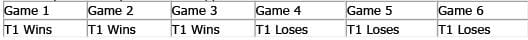There are many other ways this could happen. Using the permutation formula, there are 6!/(3!)(3!) = 20 ways for the two teams to split the first 6 games (3 wins for each).
There are then 2 possible outcomes to break the tie in Game 7. Thus, there are a total of 20 × 2 = 40 ways for the World Series to last the full 7 games.
The probability that any one of these 40 ways occurs can be calculated from the fact that the probability of a team winning a game equals the probability of a team losing a game = 1/2.
Given that 7 distinct events must happen in any 7 game series, and that each of these events has a probability of 1/2, the probability that any one particular 7 game series occurs is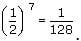Since there are 40 possible different 7 game series, the probability that the World Series will last exactly 7 games is: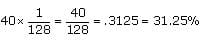Thus the probability that the World Series will last fewer than 7 games is 100% - 31.25% = 68.75%.

QUESTION: 6

Harriet and Tran each have \$10. Together, they flip a fair coin 5 times. Every time the coin lands on heads, Tran gives Harriet \$1. Every time the coin lands on tails, Harriet gives Tran \$1. After the five coin flips, what is the probability that Harriet has more than \$10 but less than \$15?

Solution:

Let's consider the different scenarios: If Harriet wins all five flips, she ends up with \$15. If Harriet wins four flips, and Tran wins one flip, Harriet is left with \$13. If Harriet wins three flips, and Tran wins two flips, Harriet is left with \$11. If Harriet wins two flips, and Tran wins three flips, Harriet is left with \$9. If Harriet wins one flip, and Tran wins four flips, Harriet is left with \$7. If Harriet loses all five flips, she ends up with \$5. The question asks for the probability that Harriet will end up with more than \$10 but less than \$15. In other words, we need to determine the probability that Harriet is left with \$11 or \$13 (since there is no way Harriet can end up with \$12 or \$14).
The probability that Harriet ends up with \$11 after the five flips: Since there are 2 possible outcomes on each flip, and there are 5 flips, the total number of possible outcomes is 2 x 2 x 2 x 2 x 2 = 32. Thus, the five flips of the coin yield 32 different outcomes. To determine the probability that Harriet will end up with \$11, we need to determine how many of these 32 outcomes include a combination of exactly three winning flips for Harriet and exactly two winning flips for Tran. This is equivalent to figuring out the possible rearrangements of THREE H’s and TWO T’s in a FIVE letter word. We can create a systematic list of combinations that include three wins for Harriet and two wins for Tran: THHHT, THHTH, THTHH, TTHHH, HTHHT, HTHTH, HTTHH, HHTHT, HHTTH, HHHTT = 10 ways. Alternatively, we can count the combinations by applying the anagram method: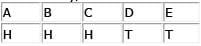We take the factorial of the top and divide by the factorial of each repeated letter on the bottom. Since there are two repeated letters, we get 5! / (3! * 2!) = 10 combinations. Thus the probability that Harriet ends up with exactly \$11 after 5 flips is 10/32. The probability that Harriet ends up with \$13 after the five flips: To determine the probability that Harriet will end up with \$13, we need to determine how many of the 32 total possible outcomes include a combination of exactly four winning flips for Harriet. Again, we can create a systematic list of combinations that include four wins for Harriet and one win for Tran: HHHHT, HHHTH, HHTHH, HTHHH, THHHH = 5 ways. Alternatively, using the same reasoning as above, we can write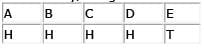The formula yields 5! / 4! = 5 combinations. Thus the probability that Harriet ends up with exactly \$13 after 5 flips is 5/32.
The total probability that Harriet ends up with either \$11 or \$13 after the five flips: There are 10 ways that Harriet is left with \$11. There are 5 ways that Harriet is left with \$13. Therefore, there are 15 ways that Harriet is left with more than \$10 but less than \$15. Since there are 32 possible outcomes, the correct answer is 15/32. Alternatively, we can observe that the two possible ways to “succeed” according to the terms of the problem are connected by a logical OR: Harriet can end up with \$11 OR \$13. When we have two avenues to success that are connected by a logical OR, we add the probabilities: 10/32 + 5/32 = 15/32.

QUESTION: 7

If 40 percent of all students at College X have brown hair and 70 percent of all students at College X have blue eyes, what is the difference between the minimum and the maximum probability of picking a student from College X who has neither brown hair nor blue eyes?

Solution:

For an overlapping set problem we can use a double-set matrix to organize our information and solve. The values here are percents, and no actual number of students is given or requested. Therefore, we can assign a value of 100 to the total number of students at College X. From the given information in the question we have: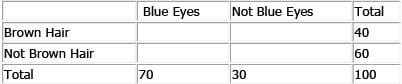The question asks for the difference between maximum value and the minimum value of the central square, that is, the percent of students who have neither brown hair nor blue eyes. The maximum value is 30, as shown below: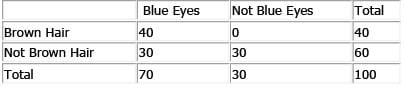Therefore the maximum probability of picking such a person is 0.3.
Likewise, the minimum value of the central square is zero, as shown below: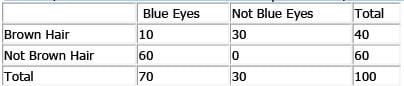Therefore the minimum probability of picking such a person is 0, and the difference between the maximum and the minimum probability is 0.3.

QUESTION: 8

In a room filled with 7 people, 4 people have exactly 1 friend in the room and 3 people have exactly 2 friends in the room (Assuming that friendship is a mutual relationship, i.e. if John is Peter's friend, Peter is John's friend). If two individuals are selected from the room at random, what is the probability that those two individuals are NOT friends?

Solution:

Begin by counting the number of relationships that exist among the 7 individuals whom we will call A, B, C, D, E, F, and G.
First consider the relationships of individual A: AB, AC, AD, AE, AF, AG = 6 total. Then consider the relationships of individual B without counting the relationship AB that was already counted before: BC, BD, BE, BF, BG = 5 total. Continuing this pattern, we can see that C will add an additional 4 relationships, D will add an additional 3 relationships, E will add an additional 2 relationships, and F will add 1 additional relationship. Thus, there are a total of 6 + 5 + 4 + 3 + 2 + 1 = 21 total relationships between the 7 individuals.
Alternatively, this can be computed formulaically as choosing a group of 2 from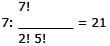We are told that 4 people have exactly 1 friend. This would account for 2 "friendship" relationships (e.g. AB and CD). We are also told that 3 people have exactly 2 friends. This would account for another 3 "friendship" relationships (e.g. EF, EG, and FG). Thus, there are 5 total "friendship" relationships in the group.
The probability that any 2 individuals in the group are friends is 5/21. The probability that any 2 individuals in the group are NOT friends = 1 – 5/21 = 16/21.

QUESTION: 9

Bill has a small deck of 12 pl aying cards made up of only 2 suits of 6 cards each.  Each of the 6 cards within a suit has a different value from 1 to 6; thus, there are 2 cards in the deck that have the same value. Bill likes to play a game in which he shuffles the deck, turns over 4 cards, and looks for pairs of cards that have the same value. What is the chance that Bill finds at least one pair of cards that have the same value?

Solution:

The chance of getting AT LEAST one pair of cards with the same value out of 4 dealt cards should be computed using the 1-x technique. That is, you should figure out the probability of getting NO PAIRS in those 4 cards (an easier probability to compute), and then subtract that probability from 1.
First card: The probability of getting NO pairs so far is 1 (since only one card has been dealt).
Second card: There is 1 card left in the deck with the same value as the first card. Thus, there are 10 cards that will NOT form a pair with the first card. We have 11 cards left in the deck.
Probability of NO pairs so far = 10/11.
Third card: Since we have gotten no pairs so far, we have two cards dealt with different values. There are 2 cards in the deck with the same values as those two cards. Thus, there are 8 cards that will not form a pair with either of those two cards. We have 10 cards left in the deck.
Probability of turning over a third card that does NOT form a pair in any way, GIVEN that we have NO pairs so far = 8/10.
Cumulative probability of avoiding a pair BOTH on the second card AND on the third card = product of the two probabilities above = (10/11) (8/10) = 8/11.
Fourth card: Now we have three cards dealt with different values. There are 3 cards in the deck with the same values; thus, there are 6 cards in the deck that will not form a pair with any of the three dealt cards. We have 9 cards left in the deck.
Probability of turning over a fourth card that does NOT form a pair in any way, GIVEN that we have NO pairs so far = 6/9.
Cumulative probability of avoiding a pair on the second card AND on the third card AND on the fourth card = cumulative product = (10/11) (8/10) (6/9) = 16/33.
Thus, the probability of getting AT LEAST ONE pair in the four cards is 1 - 16/33 = 17/33.

QUESTION: 10

If a jury of 12 people is to be selected randomly from a pool of 15 potential jurors, and the jury pool consists of 2/3 men and 1/3 women, what is the probability that the jury will comprise at least 2/3 men?

Solution:

12 people will be selected from a pool of 15 people: 10 men (2/3 of 15) and 5 women (1/3 of 15). The question asks for the probability that the jury will comprise at least 2/3 men, or at least 8 men (2/3 of 12 jurors = 8 men). The easiest way to calculate this probability is to use the “1-x shortcut.” The only way the jury will have fewer than 8 men is if a jury of 7 men and 5 women (the maximum number of women available) is selected. There cannot be fewer than 7 men on the jury, since the jury must have 12 members and only 5 women are available to serve on the jury.
The total number of juries that could be randomly selected from this jury pool is: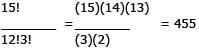The number of ways we could select 7 men from a pool of 10 men is: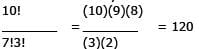The number of ways we could select 5 women from a pool of 5 women is:
5!/5! = 1
This makes practical sense, in addition to mathematical sense. All of the women would have to be on the jury, and there is only one way that can happen.
Putting these selections together, the number of ways a jury of 7 men and 5 women could be selected is: 120 × 1 = 120
The probability that the jury will be comprised of fewer than 8 men is thus 120/455 = 24/91. Therefore, the probability that the jury will be comprised of at least 8 men is 1 – (24/91) = 67/91.

QUESTION: 11

John and Peter are among the nine pl ayers a basketball coach can choose from to field a five-player team. If all five players are chosen at random, what is the probability of choosing a team that includes John and Peter?

Solution:

First we must find the total number of 5 member teams, with or without John and Peter. We can solve this using an anagram model in which each of the 9 players (A – I) is assigned either a Y (for being chosen) or an N (for not being chosen):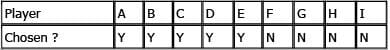It is the various arrangements of Y’s and N’s above that would yield all of the different combinations, so we can find the number of possible teams here by considering how many anagrams of YYYYYNNNN exist: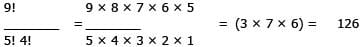(because there are 9! ways to order 9 objects) (because the 5Y's and 4N's are identical)
So there are 126 possible teams of 5. Since the question asks for the probability of choosing a team that includes John and Peter, we need to determine how many of the 126 include John and Peter. If we reserve two of the 5 spots on a team for John and Peter, there will be 3 spots left, which must be filled by 3 of the remaining 7 players (remember that John and Peter were already selected). Therefore the number of teams including John and Peter will be equal to the number of 3-player teams that can be formed from a 7-player pool. We can approach the problem as we did above: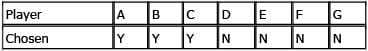The number of possible YYYNNNN anagrams is: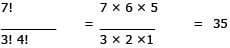Since 35 of the total possible 126 teams include John and Peter, the probability of selecting a team with both John and Peter is 35/126 or 5/18.

QUESTION: 12

A small company employs 3 men and 5 women. If a team of 4 employees is to be randomly selected to organize the company retreat, what is the probability that the team will have exactly 2 women?

Solution:

First, we must calculate the total number of possible teams (let’s call this t ). Then, we must calculate how many of these possible teams have exactly 2 women (let’s call this w). The probability that a randomly selected team will have exactly 2 women can be expressed as w/t.
To calculate the number of possible teams, we can use the Anagram Grid method. Since there are 8 employees, 4 of whom will be on the team (represented with a Y) and 4 of whom will not (represented with an N), we can arrange the following anagram grid: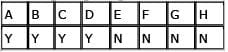To make the calculation easier, we can use the following shortcut: t = (8!)/(4!)(4!). The (8!) in the numerator comes from the fact that there are 8 total employees to choose from. The first (4!) in the denominator comes from the fact that 4 employees will be on the team, and the other (4!) comes from the fact that 4 employees will not be on the team. Simplifying yields: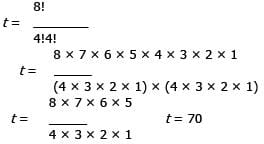So, there are 70 possible teams of 4 employees. Next, we can use a similar method to determine w, the number of possible teams with exactly 2 women. We note that in order to have exactly 2 women on the team, there must also be 2 men on the team of 4. If we calculate the number of ways that 2 out of 5 women can be selected, and the number of ways that 2 out of 3 men can be selected, we can then multiply the two to get the total number of teams consisting of 2 men and 2 women. Let’s start with the women: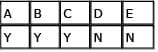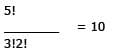So, the number of ways that 2 women can be selected is 10. Now the men: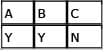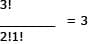Thus, the number of ways that 2 men can be selected is 3. Now we can multiply to get the total number of 2 women teams: w = (10)(3) = 30. Since there are 30 possible teams with exactly 2 women, and 70 possible teams overall, w /t = 30/70 = 3/7.

QUESTION: 13

A box contains one dozen donuts. Four of the donuts are chocolate, four are glazed, and four are jelly. If two donuts are randomly selected from the box, one after the other, what is the probability that both will be jelly donuts?

Solution:

To determine the probability that jelly donuts will be chosen on the first and second selections, we must find the probability of both events and multiply them together. The probability of picking a jelly donut on the first pick is 4/12. However, the probability of picking a jelly donut on the second pick is NOT 4/12. If a jelly donut is selected on the first pick, the number of donuts in the box has decreased from 12 to 11, and the number of jelly donuts has decreased from 4 to 3. Thus, the probability of picking a jelly donut on the second pick is 3/11.
Since overall probability is calculated by multiplying the probabilities of both events, the probability of picking two jelly donuts is 4/12 × 3/11 = 12/132 = 1/11.

QUESTION: 14

A hand purse contains 6 nickels, 5 pennies and 4 dimes. What is the probability of picking a coin other than a nickel twice in a row if the first coin picked is not put back?

Solution:

To find the probability that two independent events will occur, one after the other, multiply the probability of the first event by the probability of the second event. Probability of a non-nickel on first pick = (5 pennies + 4 dimes) / 15 coins = 3/5 Probability of a non-nickel on second pick = (8 non-nickel coins) / 14 coins = 4/7 Notice that for the second pick both the non-nickel pool and the total coin pool diminished by one coin after a nonnickel was selected on the first pick.
Total probability = 3/5 x 4/7 = 12/35.

QUESTION: 15

Jim and Renee will play one game of Rock, Paper, Scissors. In this game, each will select and show a hand sign for one of the three items. Rock beats Scissors, Scissors beat Paper, and Paper beats Rock. Assuming that both Jim and Renee have an equal chance of choosing any one of the hand signs, what is the probability that Jim will win?

Solution:

No matter what sign Jim throws, there is one sign Renee could throw that would beat it, one that would tie, and one that would lose. Renee is equally likely to throw any one of the three signs. Therefore, the probability that Jim will win is 1/3.
For example, Jim could throw a Rock sign. He will win only if Renee throws a Scissors sign. There is a one in three chance that Renee will do so.
In fact: Probability that Renee will win = 1/3 Probability of a tie = 1/3
Probability that Jim will win = 1/3Use Code STAYHOME200 and get INR 200 additional OFF Use Coupon Code